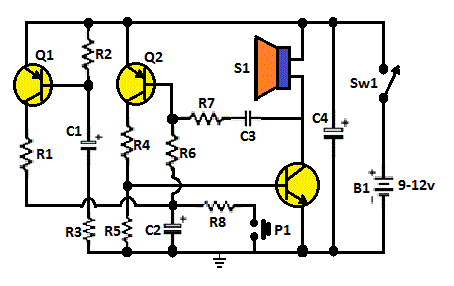Search

# L34, Powerful Security SirenThis circuit is configured by several components. Its purpose was to obtain more power than the simple siren circuit. A complementary transistor pair (Q2 & Q3) is wired as a high efficiency oscillator, directly driving the loudspeaker. Q1 ensures a full charge of C2 when power is applied to the circuit. Pressing on P1, C2 gradually discharges through R8, the circuit starts oscillating at a low frequency that increases slowly until a high steady tone is reached and kept indefinitely. When P1 is released, the output tone frequency decreases slowly as C2 is charged to the battery positive voltage through R6 and the Base-Emitter junction of Q2. When C2 is fully charged the circuit stops oscillating, reaching a stand-by status.

Parts:

• R1 = 1K, R2 = 10K, R3 = 1K, R4 = 220R

• R5 = 10K, R6= 220K, R7 = 22K, R8 = 100K

• C1 = 22uF-25V, C2 = 22uF-25V, C3 = 10nF-63V, C4 = 47uF-25V

• Q1 = BC557,

• Q2 = BC557

• Q3 = BC337

• P1 = Pushbutton

• B1 = -9-12V Battery

• SW1 = SPST Toggle or Slide Main Switch

• SPKR = 8 Ohms Loudspeaker

Notes:

• A good sized loudspeaker will ensure a better and powerful output tone.

• As stand-by current drawing is zero, SW1 can be omitted and B1 wired directly to the circuit.

• Maximum current drawing at full output is about 200mA.